# How to Control Stepper Motor using Potentiometer and Arduino

Published  August 21, 2017   3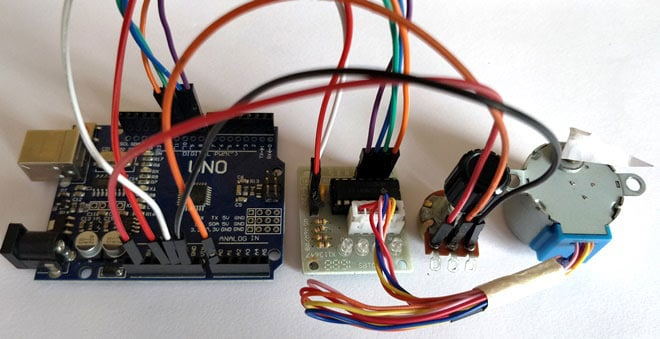Stepper motors are increasingly taking its position in the world of the electronics. Starting from a normal Surveillance camera to a complicated CNC machines/Robot these Stepper Motors are used everywhere as actuators since they provide accurate controlling. In this tutorial we will learn about the most commonly/cheaply available stepper motor 28-BYJ48 and how to interface it with Arduino using ULN2003 stepper module.

In last project we have simply Interfaced Stepper Motor with Arduino, where you can rotate the stepper motor by entering the rotation angle in Serial Monitor of Arduino. Here in this project, we will Rotate the Stepper Motor using Potentiometer and Arduino, like if you turn the potentiometer clockwise then stepper will rotate clockwise and if you turn potentiometer anticlockwise then it will rotate anticlockwise.

### Stepper Motors:

Let us take a look at this 28-BYJ48 Stepper motor.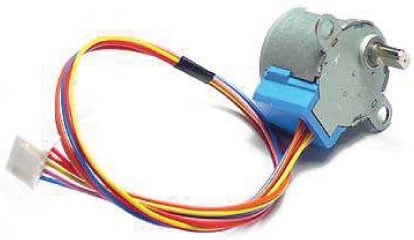Okay, so unlike a normal DC motor this one has five wires of all fancy colors coming out of it and why is it so? To understand this we should first know how a stepper works and what its specialty is. First of all steppers motors do not rotate, they step and so they also known as step motors. Meaning, they will move only one step at a time. These motors have a sequence of coils present in them and these coils have to be energized in a particular fashion to make the motor rotate. When each coil is being energized the motor takes a step and a sequence of energization will make the motor take continuous steps, thus making it to rotate. Let us take a look at the coils present inside the motor to know exactly know from where these wires come from.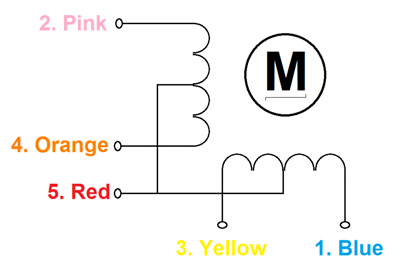As you can see the motor has Unipolar 5-lead coil arrangement. There are four coils which have to be energized in a particular sequence. The Red wires will be supplied with +5V and the remaining four wires will be pulled to ground for triggering the respective coil. We use a microcontroller like Arduino energize these coils in a particular sequence and make the motor perform the required number of steps.

So now, why is this motor called the 28-BYJ48? Seriously!!! I don’t know. There is no technical reason for this motor for being named so; maybe we should dive much deeper into it. Let us look at some of the important technical data obtained from the datasheet of this motor in the picture below.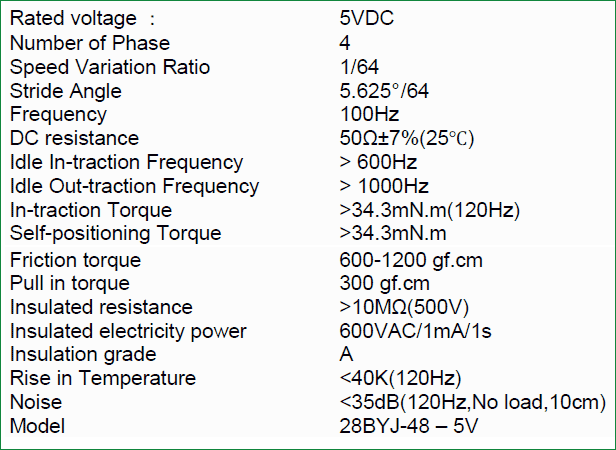That is a head full of information, but we need to look at few important ones to know what type of stepper we are using so that we can program it efficiently. First we know that it is a 5V Stepper motor since we energize the Red wire with 5V. Then, we also know that it is a four phase stepper motor since it had four coils in it. Now, the gear ratio is given to be 1:64. This means the shaft that you see outside will make one complete rotation only if the motor inside rotates for 64 times. This is because of the gears that are connected between the motor and output shaft, these gears help in increasing the torque.

Another important data to notice is the Stride Angle: 5.625°/64. This means that the motor when operates in 8-step sequence will move 5.625 degree for each step and it will take 64 steps (5.625*64=360) to complete one full rotation.

### Calculating the Steps per Revolution for Stepper Motor:

It is important to know how to calculate the steps per Revolution for your stepper motor because only then you can program it effectively.

In Arduino we will be operating the motor in 4-step sequence so the stride angle will be 11.25° since it is 5.625°(given in datasheet) for 8 step  sequence it will be 11.25° (5.625*2=11.25).

Steps per revolution = 360/step angle

Here, 360/11.25 = 32 steps per revolution.

### Why so we need Driver modules for Stepper motors?

Most stepper motors will operate only with the help of a driver module. This is because the controller module (In our case Arduino) will not be able to provide enough current from its I/O pins for the motor to operate. So we will use an external module like ULN2003 module as stepper motor driver. There are a many types of driver module and the rating of one will change based on the type of motor used. The primary principle for all driver modules will be to source/sink enough current for the motor to operate.

### Circuit Diagram for Rotating Stepper Motor using Potentiometer: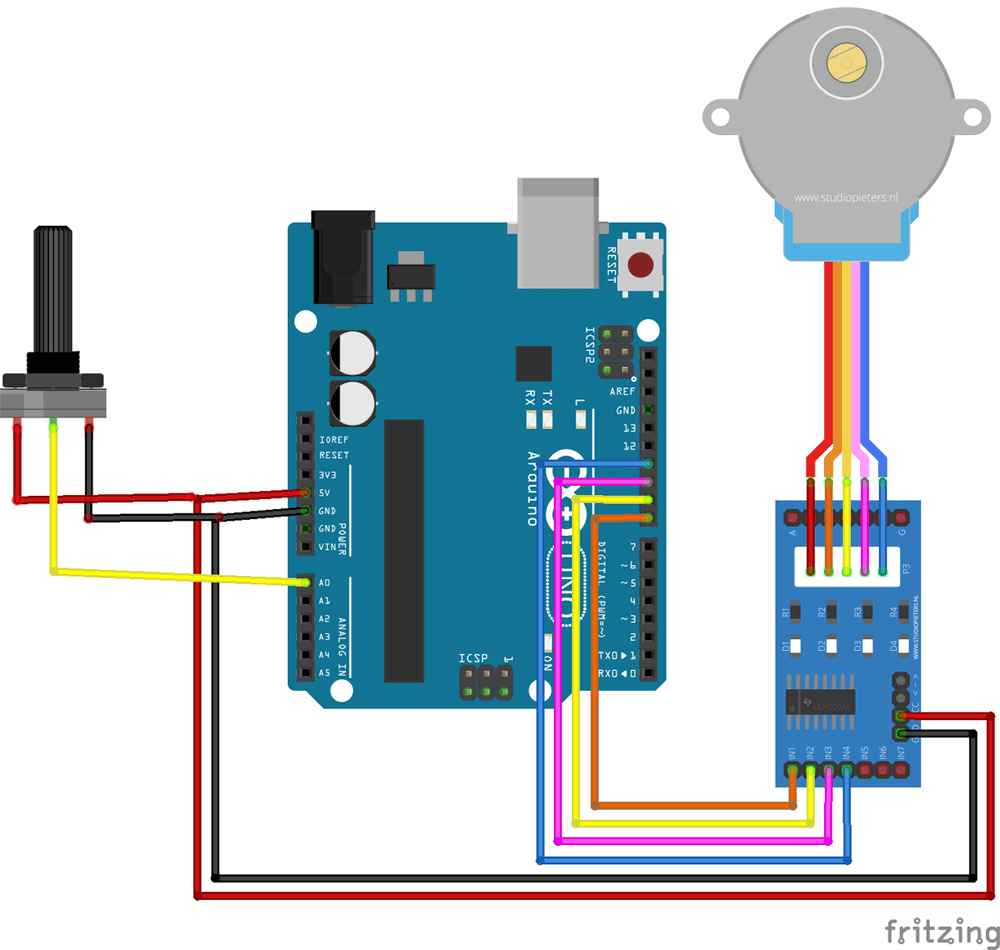The circuit Diagram for the Controlling Stepper Motor using Potentiometer and Arduino is shown above. We have used the 28BYJ-48 Stepper motor and the ULN2003 Driver module. To energise the four coils of the stepper motor we are using the digital pins 8,9,10 and 11. The driver module is powered by the 5V pin of the Arduino Board. A potentiometer is connected to A0 based in whose values we will rotate the Stepper motor.

But, power the driver with External Power supply when you are connecting some load to the steppe motor. Since I am just using the motor for demonstration purpose I have used the +5V rail of the Arduino Board. Also remember to connect the Ground of the Arduino with the ground of the Driver module.

### Code for Arduino Board:

Before we start programming with our Arduino, let us understand what should actually happen inside the program. As said earlier we will be using 4-step sequence method so we will have four steps to perform for making one complete rotation.

 Step Pin Energized Coils Energized Step 1 8 and 9 A and B Step 2 9 and 10 B and C Step 3 10 and 11 C and D Step 4 11 and 8 D and A

The Driver module will have four LED using which we can check which coil is being energised at any given time. The complete demonstration video  can be found at the end of this tutorial.

In this tutorial we are going to program the Arduino in such a way that we can turn the potentiometer connected to pin A0 and control the direction of the Stepper motor. The complete program can be found at the end of the tutorial few important lines are explained below.

The number of steps per revolution for our stepper motor was calculated to be 32; hence we enter that as shown in the line below

`#define STEPS 32`

Next you have to create instances in which we specify the pins to which we have connected the Stepper motor.

`Stepper stepper (STEPS, 8, 10, 9, 11);`

Note: The pins number are disordered as 8,10,9,11 on purpose. You have to follow the same pattern even if you change the pins to which your motor is connected.

Since we are using the Arduino stepper library, we can set the speed of the motor using the below line. The speed can range between 0 to 200 for 28-BYJ48 stepper motors.

`stepper.setSpeed(200);`

Now, to make the motor move one step clockwise we can use the following line.

`stepper.step(1);`

To make the motor move one step anti-clockwise we can use the following line.

`stepper.step(-1);`

In our program we will read the value of the Analog pin A0 and compare it with previous value (Pval). If it has increased we move 5 steps in clockwise and if it is decreased then we move 5 steps in anti-clockwise.

```potVal = map(analogRead(A0),0,1024,0,500);
if (potVal>Pval)
stepper.step(5);
if (potVal<Pval)
stepper.step(-5);
Pval = potVal;```

### Working:

Once the connection is made the hardware should look something like this in the picture below.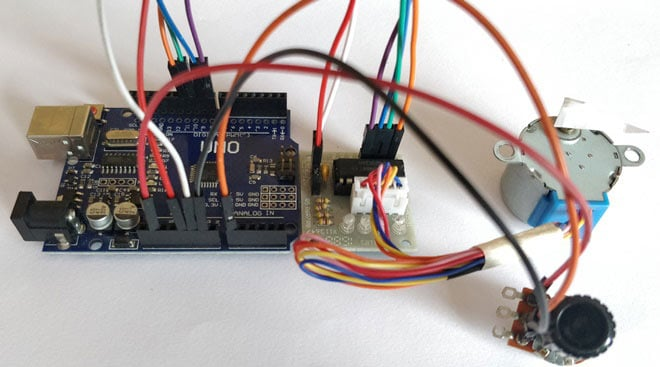Now, upload the below program in your Arduino UNO and open the serial monitor. As discussed earlier you have to rotate the potentiometer to control the rotation of the Stepper motor. Rotating it in clockwise will turn the stepper motor in clockwise direction and vice versa.

Hope you understood the project and enjoyed building it. The complete working of the project is shown in the video below. If you have any doubts post them on the comment section below or on our forums.

Code

#include <Stepper.h> // Include the header file

// change this to the number of steps on your motor
#define STEPS 32

// create an instance of the stepper class using the steps and pins
Stepper stepper(STEPS, 8, 10, 9, 11);

int Pval = 0;
int potVal = 0;

void setup() {
Serial.begin(9600);
stepper.setSpeed(200);
}

void loop() {

if (potVal>Pval)
stepper.step(5);
if (potVal<Pval)
stepper.step(-5);

Pval = potVal;

Serial.println(Pval); //for debugging
}

Video

Tags